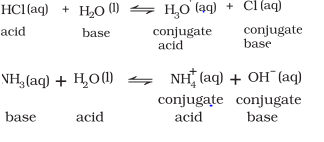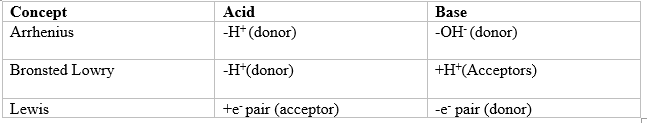# Le Chatelier's Principle

## Acid, Bases and Salt

• We can find Acid, Bases and Salt in many areas in our life.
• Acids are known to turn blue litmus paper into red and liberate dihydrogen on reacting with some metals.
• Bases are known to turn red litmus paper blue, taste bitter and feel soapy.Example is washing soda used for washing purposes.
• When acids and bases are mixed in the right proportion they react with each other to give salts Examples: sodium chloride, barium sulphate

## Arrhenius Concept of Acids and Bases

Acids are $H^+$ donors while bases are OH donar. Eg, $H_2SO_4$ is acid, NaOH is base.
Example Of Acid
The ionization of an acid HX (aq) can be represented by the following equations:
$HX (aq) -> H^+(aq) + X^– (aq)$
or
$HX(aq) + H_2O(l) -> H_3O^+(aq) + X^–(aq)$
Example Of Base
The ionization of an base is represented by the below equation
$MOH(aq) → M^+(aq) + OH^–(aq)$
Limitations of Arrhenius concept
It doesn’t give any information about basity of compounds like $NH_3$ not having OH groups.

### The Bronsted-Lowry Acids and Bases

• Acids are $H^+$ donars while bases are $H^+$ acceptors.
• acids are proton donors and bases are proton acceptor
• The acid-base pair that differs only by one proton is called a conjugate acid-base pair.
Eg:-## Lewis Acids and Bases

• Lewis acids are $e^-$ pair acceptor while Lewis bases are $e^-$ pair donors.
• Lewis acids are $e^-$ deficient compounds. Eg:- $Mg^{+2}$,$AlCl_3$,$BF_3$, $BCl_3$, etc.
• Lewis bases are $e^-$ rich species. Eg:- $OH^-$, $Cl^-$, $NH_3$, $H_2O$

## Comparison between Arrhenius,Bronsted-Lowry and Lewis## Examples

1.Conjugate acid and base of $HNO_2$
$H_2NO_2^+$ is the conjugate acid
$NO_2^-$ is the conjugate Base

2.Is $BCl_3$ a Lewis Acid or Lewis Base
$BCl_3$ acts as a Lewis acid as it can accept a lone pair of electrons from species like ammonia or amine molecules.